# Tractors

Fields go plow two tractors with various performance. The first tractor plow whole field in 11 hours, the second tractor plow whole field 19 hours longer.

How long take plow whole field with two tractors?

Result

t =  8.049 h

#### Solution:

t*(1/11 + 1/30) = 1

41t = 330

t = 33041 ≈ 8.04878

Calculated by our simple equation calculator.

Leave us a comment of example and its solution (i.e. if it is still somewhat unclear...):

Showing 0 comments:Be the first to comment!#### To solve this example are needed these knowledge from mathematics:

Looking for help with calculating harmonic mean? Looking for a statistical calculator? Need help calculate sum, simplify or multiply fractions? Try our fraction calculator.

## Next similar examples:

1. Red diplomasHe numbers of students with honors in 2013 and 2014 are in ratio 40:49. How big is the year-on-year percentage increase?
2. Eq with reciprocalSolve given equation with reciprocal member: a-6/a+10=4/8
3. BW-BS balls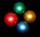Adam has a full box of balls that are large or small, black or white. Ratio of large and small balls is 5:3. Within the large balls the ratio of the black to white is 1:2 and between small balls the ratio of the black to white is 1:8 What is the ratio of.
4. Hectoliters of waterThe pool has a total of 126 hectoliters of water. The first pump draws 2.1 liters of water per second. A second pump pumps 3.5 liters of water per second. How long will it take both pumps to drain four-fifths of the water at the same time?
5. Two pumps togetherThe first pump will fill the tank itself in 3 hours and the second one in 6 hours. How many hours will the tank be full if both pumps are worked at the same time?
6. Theorem proveWe want to prove the sentense: If the natural number n is divisible by six, then n is divisible by three. From what assumption we started?
7. Painter Pavel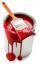Painter Pavel painted the fence for 16 hours and painter Petr the same fence painted for 13 h. How long should take painted the fence together?
8. Minute averageIn a factory, four workers are assigned to complete an order received for dispatching 1400 boxes of a particular commodity. Worker A takes 4 mins per box, Worker B takes 6 minutes per box, C takes 10 mins per box, D takes 15 mins per box. Find the average.
9. Work at plant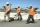Handyman has vowed to do repair work at the plant for 25 days. However, work had to be shortened and therefore gained helper. Together they made all the corrections for 13 1/3 days. How long the work would take helper himself?
10. Automaker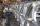The automaker now produces daily 4 new cars more than last year so the production of 360 cars will save just one full working day. How many working days to produce 360 vehicles needed last year?
11. Cyclist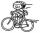Cyclist goes uphill 10 km for 50 minutes and downhill minutes for 29 minutes, both applied to the pedals same force. How long he pass 10 km by plane?
12. CandiesIf Alena give Lenka 3 candy will still have 1 more candy. If Lenka give Alena 1 candy Alena will hame twice more than Lenka. How many candies have each of them?
13. 5 pumps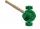Five pump to pump 3 hours 1600 hl of water. How long pumped same amount of water 4 pumps?
14. The diceWhat is the probability of events that if we throw a dice is rolled less than 6?
15. AntennasIf you give me two antennas will be same. If you give me again your two antenna I have a 5× so many than you. How many antennas have both mans?
16. Liquid soap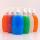Liquid soap lasted two weeks to one member of the family. How many days will last, if it use: a) two members b) 4 members c) seven members?
17. Workers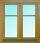Group of workers washing half of the windows of the building in two hours. How long will take half the workers wash all the windows of the building?